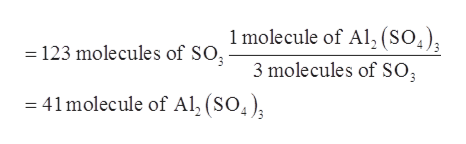# Based on the balanced equation, Al2O3 + 3SO3 → Al2(SO4)3 calculate the formula units of Al2(SO4)3 formed when 123 molecules of SO3 are reacted.

Question
27 views

Based on the balanced equation,

Al2O3 + 3SO3 → Al2(SO4)3

calculate the formula units of Al2(SO4)3 formed when 123 molecules of SO3 are reacted.

check_circle

Step 1

The molecules that present inside any substance can be determined by calculating the number of moles. These moles are then multiplied by the Avogadro’s number to get the molecules present in the substance.

Step 2

The given balanced reaction is shown below.

Step 3

From the above balanced reaction, it is clear that one molecule of Al2(SO4)3 is produced by 3 molecules of SO3. Therefore, the number of molecules of Al...help_outlineImage Transcriptionclose1 molecule of Al, (SO4) 3 molecules of SO 123 molecules of SOg - = 41molecule of Al, (SO4) fullscreen

### Want to see the full answer?

See Solution

#### Want to see this answer and more?

Solutions are written by subject experts who are available 24/7. Questions are typically answered within 1 hour.*

See Solution
*Response times may vary by subject and question.
Tagged in

### Chemistry